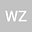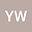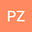Multiplicity and concentration of positive solutions for quasilinear Schrödinger-Poisson system with critical nonlinearity
•••yanyun wen
University of New South Wales School of Mathematics and Statistics
Author ProfilePeihao Zhao
University of New South Wales School of Mathematics and Statistics
Author Profile## Abstract

In this paper, we are interested in the following Schrödinger-Poisson system { − ε p ∆ p u + V ( x ) | u | p − 2 u + ϕ | u | p − 2 u = f ( u )+ | u | p ∗ − 2 u in R 3 , − ε 2 ∆ ϕ = | u | p in R 3 , where ε>0 is a parameter, 3 2 < p < 3 , ∆ p u = div ( | ∇ u | p − 2 ∇ u ) , p ∗ = 3 p 3 − p , V : R 3 → R is a positive function with a local minimum and f is subcritical growth. Based on the penalization method, Nehari manifold techniques and Ljusternik-Schnirelmann category theory, we obtain the multiplicity and concentration of positive solutions.